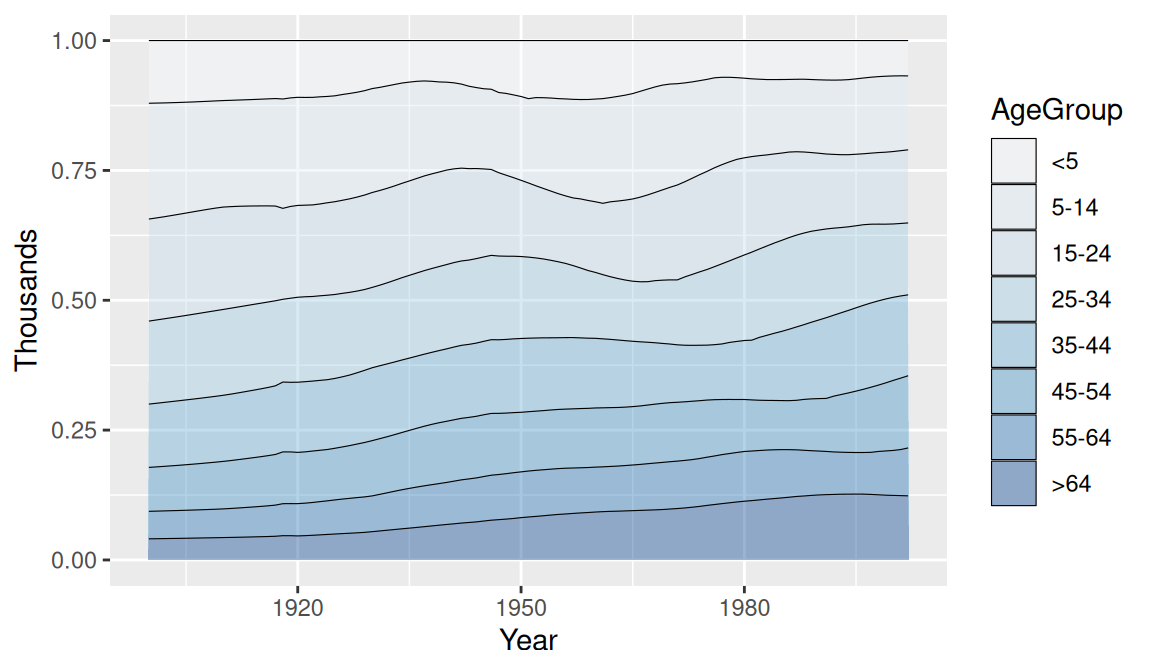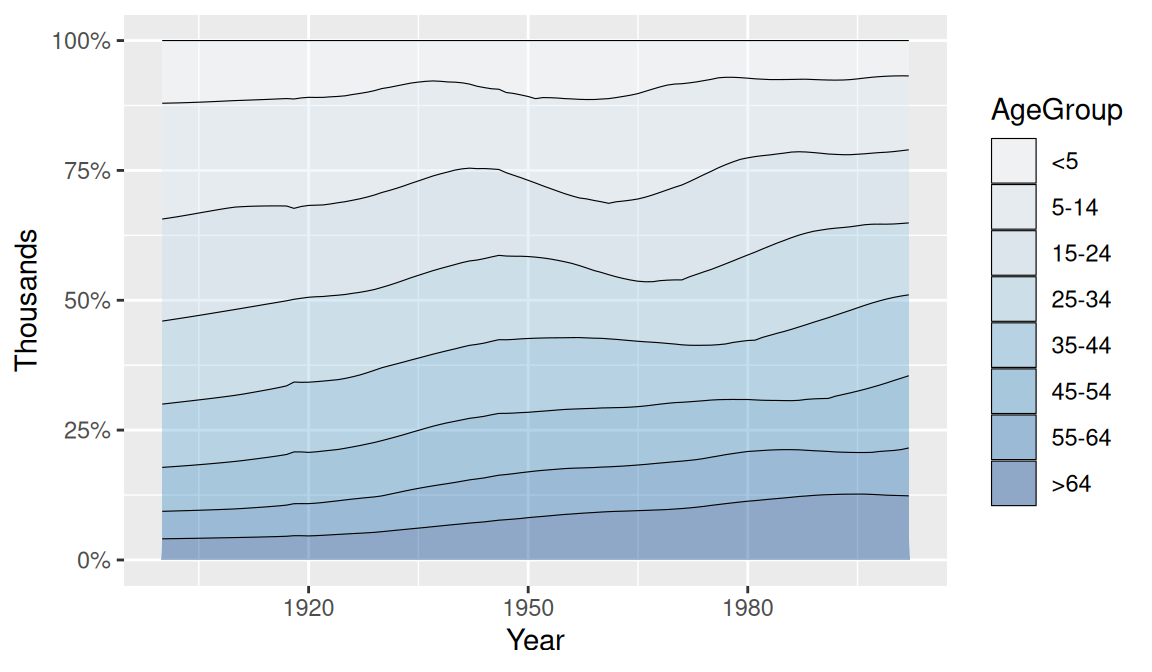## 4.8 Making a Proportional Stacked Area Graph

### 4.8.1 Problem

You want to make a stacked area graph with the overall height scaled to a constant value.

### 4.8.2 Solution

Use `geom_area(position = "fill")`, as in Figure 4.23, left:

``````ggplot(uspopage, aes(x = Year, y = Thousands, fill = AgeGroup)) +
geom_area(position = "fill", colour = "black", size = .2, alpha = .4) +
scale_fill_brewer(palette = "Blues")``````

### 4.8.3 Discussion

With `position="fill"`, the y values will be scaled to go from 0 to 1. To print the labels as percentages, use `scale_y_continuous(labels = scales::percent)`, as in Figure 4.23, right:

``````ggplot(uspopage, aes(x = Year, y = Thousands, fill = AgeGroup)) +
geom_area(position = "fill", colour = "black", size = .2, alpha = .4) +
scale_fill_brewer(palette = "Blues") +
scale_y_continuous(labels = scales::percent)``````Figure 4.23: A proportional stacked area graph